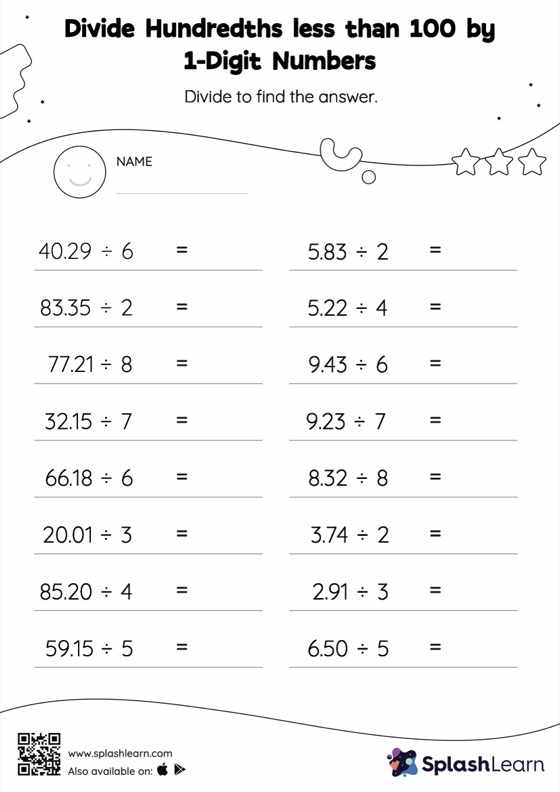# Divide Hundredths less than 100 by 1-Digit Numbers: Horizontal Division Worksheet

Home > Divide Hundredths less than 100 by 1-Digit Numbers: Horizontal DivisionStudents begin by dividing decimals by 1-digit integers in the same manner they would with whole numbers. Depending on the number of decimal digits in the dividend, they add a decimal point at the appropriate location in the quotient. They get more confidence with this concept thanks to divide hundredths less than 100 by 1-digit numbers worksheet. As the worksheet uses a horizontal format, it allows for more creativity when it comes to how the student solves the problem. In contrast, in the vertical format, students usually employ the standard method to solve.